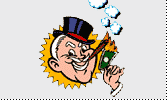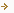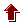##### All Trivia Game - Facts and Trivia DirectoryHomeTrivia and factsTrivia gameLinksMath Facts

A 'jiffy' is an actual unit of time for 1/100th of a second.

1 and 2 are the only numbers where they are the values of the numbers of factors they have.

'Forty' is the only number that has all its letters in alphabetical order.

'FOUR' is the only number in the English language that is spelt with the same number of letters as the number itself

Multiply 37,037 by any single number (1-9), then multiply that number by 3. Every digit in the answer will be the same as that first single number.

1x9 +2 = 11 , 12x9 +3 = 111 , 123x9+4 = 1111 etc

2 and 5 are the only primes that end in 2 or 5.

The 772-778 digits of pi are 9999998, the greatest sum of 7 consecutive digits in the first 1,000,000 or so digits.

There are 2,598,960 five-card hands possible in a 52-card deck of cards.

The largest prime number is 13,395 digits long; more than the number of atoms in the universe.

142857 is a cyclic number, the numbers of which always appear in the same order but rotated around when multipled by any number from 1 to 6. 142857 * 2 = 285714 142857 * 3 = 428571 142857 * 4 = 571428 142857 * 5 = 714285 142857 * 6 = 857142

Tycho Brahe, a 16th century astronomer, lost his nose in a duel with one of his students over a mathematical computation. He wore a silver replacement nose for the rest of his life.

If you multiply 1089 x 9 you get the exact reverse, 9801.

 Back to indexSubmit math facts and triviaAllTrivia.net © 2002-2006 All Trivia Game, Claremont California, 91711